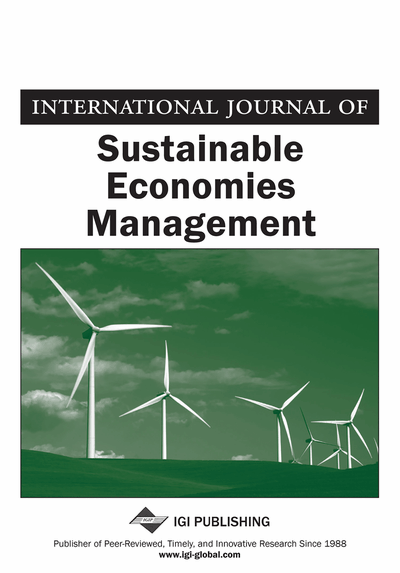# A Multivariable System Approach for the Assessment of the Influence of Fiscal Policy to Business Cycle Shocks

Iraklis Kollias, John Leventides
DOI: 10.4018/ijsem.2013040103
OnDemand:
(Individual Articles)
Available
\$29.50
No Current Special Offers

## Abstract

The authors consider a multivariable system comprising a set of macroeconomic variables as inputs, states and outputs. In this setting the authors assess the control potential of fiscal policy versus a large negative business cycle shock that affects the output of the system. The inputs considered for this matter are the cyclical components of government spending and taxes. The states are the cyclical components of consumption and investment. These variables convey information to the policy maker at each time period. The output of the system is the cyclical component of GDP. In order to assess the control potential of government fiscal policy we begin by solving an optimal control problem minimizing the deviations of log GDP from its trend subject to a multivariable system and a set of input - output inequality constraints. Based on the results obtained the authors propose a set of optimal control indicators used to evaluate the effectiveness of fiscal policy action against a large negative business cycle shock and the potential controllability of the system.
Article Preview
Top

## 1. Introduction

Since Sims's (1980) criticism of large - scale statistical macroeconomic models for assuming “incredible” identifying restrictions, the use of vector autoregressive (VAR) models over the past three decades has grown more and more popular among empirical macroeconomists and policy making economists alike. VAR models have been used extensively in monetary economics to study the effects of monetary shocks on both money and real variables such as employment and real output (see e.g. Eichenbaum et al., 1998 and references therein). More recently they have also been used to study the effects of fiscal policy (see e.g. Blanchard & Perotti, 2002, Perotti et al., 2007).

A VAR model is a stochastic linear time - invariant vector difference equation and may be considered as the reduced form of a system of structural equations in which there are no exogenous variables but only lagged endogenous variables. As is well known, in order to perform forecasts using an econometric model of equations, at least in the short run, the knowledge of the structural parameters is not a prerequisite. Only the reduced form parameters are required whose estimation is always feasible using ordinary least squares (OLS). It is precisely the conduct of short run forecasts for which VAR models have been used extensively. From the point of view of control theory, a VAR model is termed as uncontrolled since there is no input that can be selected in order to control the output of the system. In each case a shock occurs to some variable(s) and from that point onwards the system's response is determined by its uncontrolled dynamics.

In this paper we begin by formulating a control system in the form of a VAR model. The control variables considered are the cyclical components of government spending and taxes. The state variables are private consumption and investment. The output of the VAR control system is the GDP. All variables are modeled as deviations from the trend. The decomposition between the cyclical part and the trend may be realized by a Hodrick - Prescott filter or by other detrending methods (see Canova, 1998). After estimating the controlled vector autoregressive model (henceforth, C - VAR) we solve the optimal control problem of minimizing the effect of business cycle shocks via shaping the cyclical components of government expenditure and taxes. The solution is based on the following two assumptions: (i) A large negative shock to consumption and investment equal to twice the minimum value of the residuals distributions of these variables and (ii) two inequality constraints of the form,, where the constantsandare appropriately selected. The objective functional considered is, wheredenotes the deviation of the GDP from the trend at time. The aim is to minimize this functional subject to the C - VAR model and the inequality constraints and thus minimize the effect of the business cycle shock.

The control schemes considered are both open - loop and closed - loop. In the first case, an open - loop optimal control is to be determined such that the objective functional is minimized subject to the constraints of the optimal control problem. In the latter case, a dynamic state feedback controller is designed such that the objective functional is minimized subject to compensated dynamics and the inequality constraints. The solution to the optimal control problem, both open - loop and closed - loop, will allow us to evaluate a set of economies based on a variety of indices such as minimum value of the objective functional, i.e. size of error, poles of the C - VAR model, percentage error reduction when feedback is applied and pole magnitude reduction among other.

## Complete Article List

Search this Journal:
Reset
Volume 12: 1 Issue (2023): Forthcoming, Available for Pre-Order
Volume 11: 4 Issues (2022): 1 Released, 3 Forthcoming
Volume 10: 4 Issues (2021)
Volume 9: 4 Issues (2020)
Volume 8: 4 Issues (2019)
Volume 7: 4 Issues (2018)
Volume 6: 4 Issues (2017)
Volume 5: 4 Issues (2016)
Volume 4: 4 Issues (2015)
Volume 3: 4 Issues (2014)
Volume 2: 4 Issues (2013)
Volume 1: 4 Issues (2012)
View Complete Journal Contents Listing